Test Description

## 20 Questions MCQ Test Math Olympiad for Class 5 | Olympiad Test: Temperature

Olympiad Test: Temperature for Class 5 2023 is part of Math Olympiad for Class 5 preparation. The Olympiad Test: Temperature questions and answers have been prepared according to the Class 5 exam syllabus.The Olympiad Test: Temperature MCQs are made for Class 5 2023 Exam. Find important definitions, questions, notes, meanings, examples, exercises, MCQs and online tests for Olympiad Test: Temperature below.
 1 Crore+ students have signed up on EduRev. Have you?
Olympiad Test: Temperature - Question 1

### Ten celsius equals _?_farenheit.

Olympiad Test: Temperature - Question 2

### Convert 32° Celsius to Fahrenheit.

Detailed Solution for Olympiad Test: Temperature - Question 2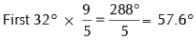Then: 57.6° + 32° = 89.6°F

Olympiad Test: Temperature - Question 3

### Eighty Fahrenheit is _?_ Celsius.

Olympiad Test: Temperature - Question 4

Which is the formula to convert Celsius to Fahrenheit?

Olympiad Test: Temperature - Question 5

Convert 11° Celsius to Fahrenheit.

Detailed Solution for Olympiad Test: Temperature - Question 5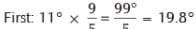Then: 19.8° + 32° = 51.8°F

Olympiad Test: Temperature - Question 6

Convert 68° Fahrenheit to Celsius.

Detailed Solution for Olympiad Test: Temperature - Question 6

First: 68° – 32° = 36°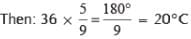Olympiad Test: Temperature - Question 7

Convert 18° Fahrenheit to Celsius.

Detailed Solution for Olympiad Test: Temperature - Question 7

First: 18° – 32° = –14°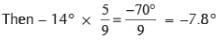(to 1 dec place)

Olympiad Test: Temperature - Question 8

The highest temperature ever recorded on Earth was 57.8°C, in Libya, Africa in 1922.How many degrees Fahrenheit was this, to the nearest degree?

Detailed Solution for Olympiad Test: Temperature - Question 8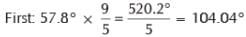Then: 104.04° + 32° = 136.04°F.
This is 136°F to the nearest degree

Olympiad Test: Temperature - Question 9

The highest temperature ever recorded in the UK was 101.3°F, recorded in Kent in 2003.

Detailed Solution for Olympiad Test: Temperature - Question 9

First: 101.3° – 32° = 69.3°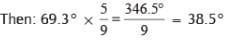Olympiad Test: Temperature - Question 10

Convert 41° Fahrenheit to Celsius:

Detailed Solution for Olympiad Test: Temperature - Question 10

(41 °F – 32) × (5/9)
=9 × (5/9) = 5 ° C

Olympiad Test: Temperature - Question 11

The lowest temperature ever recorded on Earth was – 89.2°C, recorded in Antarctica in 1983. How many degrees Fahrenheit was that, to the nearest degree?

Detailed Solution for Olympiad Test: Temperature - Question 11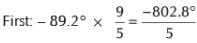= – 160.56°
Then: –160.56° + 32° = –128.56°F
which is –129°F to the nearest degree.

Olympiad Test: Temperature - Question 12

Convert 13° Fahrenheit to Celsius:

Detailed Solution for Olympiad Test: Temperature - Question 12

(13 ° F – 32) × (5/9)
= – 19 × (5/9)
= – 10.6 ° C

Olympiad Test: Temperature - Question 13

The fastest temperature drop ever recorded was 49°F in 15 minutes, recorded in Rapid City, South Dakota, USA in 1911. How many degrees Celsius was this?

Detailed Solution for Olympiad Test: Temperature - Question 13

Note that these are temperature changes, not actual temperatures, so we do not first subtract 32°. To change from degrees Fahrenheit to degrees Celsius, we just multiply by 5/9:

Olympiad Test: Temperature - Question 14

What is the formula to convert to fahrenheit to celsius?

Olympiad Test: Temperature - Question 15

Convert 100° Celsius to Fahrenheit

Detailed Solution for Olympiad Test: Temperature - Question 15

100° C × (9/5) + 32
= 180 + 32
= 212° F

Olympiad Test: Temperature - Question 16

Convert 19° Celsius to Fahrenheit

Detailed Solution for Olympiad Test: Temperature - Question 16

19° C × (9/5) + 32
= 34.2 + 32
= 66.2° F

Olympiad Test: Temperature - Question 17

On the Fahrenheit scale, the freezing point of water is _____ degrees and the boiling point is ____ degrees.

Olympiad Test: Temperature - Question 18

The Celsius temperature scale is still sometimes referred to as the _?_ scale.

Olympiad Test: Temperature - Question 19

Convert 14° Celsius to Fahrenheit.

Detailed Solution for Olympiad Test: Temperature - Question 19

14°C × (9/5) + 32 = 25.2 + 32
=57.2°F

Olympiad Test: Temperature - Question 20

Convert 73° Fahrenheit to Celsius.

Detailed Solution for Olympiad Test: Temperature - Question 20

(73 ° F –32) × (5/9)
= 41 × (5/9)
= 22.8 ° C

## Math Olympiad for Class 5

2 videos|3 docs|40 tests
 Use Code STAYHOME200 and get INR 200 additional OFF Use Coupon Code